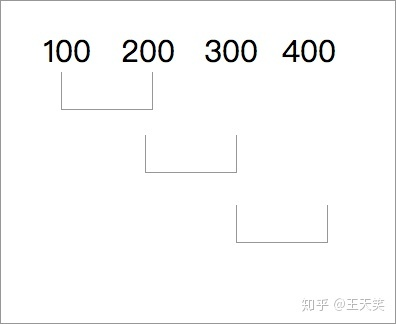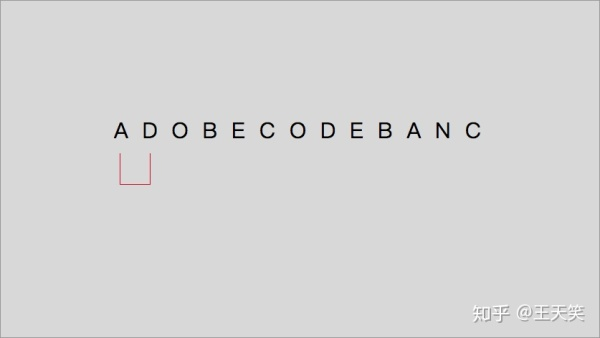• 滑动窗口算法求最大无重复子串长度： ** 1.维护一个起始长度为0的窗口，窗口内都是没有重复的字符。 2.逐个遍历接收到的字符串，如果新遍历到的字符没有在窗口中出现过，那么窗口就“吃掉”这个字符，窗口右边...
滑动窗口算法求最大无重复子串长度：
**

1.维护一个起始长度为0的窗口，窗口内都是没有重复的字符。
2.逐个遍历接收到的字符串，如果新遍历到的字符没有在窗口中出现过，那么窗口就“吃掉”这个字符，窗口右边界索引+1，左边界保持不变。
3.如果连续遍历到的字符都没有出现在窗口中，那么窗口将连续扩大。
4.如果遍历的字符在窗口中出现过，那么左窗口向右移动。
5.持续进行遍历，直到最后一个字符。
6.窗口只会扩大，不会缩小，每次记录窗口的最大值。

**
话不多说，来人，上代码！
def lengthoflongestsubstring(s):
dic,maxln,start_index={},0,0
all_str=[]
for index,item in enumerate(s):
if item in dic:     #如果窗口中存在当前字符，则左边界向右移动
start_index=max(start_index,dic[item]+1)
maxln=max(maxln,index-start_index+1)   #如果不存在，则扩大窗口
windows=s[index +1- maxln:index+1]   #得到窗口中的子串
all_str.append(windows)  #记录所有子串到列表
dic[item] = index
#print("所有子窗口为%s" %all_str)
unexpect_str=[]
for obj in all_str:
for i in range(len(obj)):
if obj.count(obj[i])!=1 or len(obj)<maxln:
unexpect_str.append(obj)
for j in unexpect_str:
if j in all_str:
all_str.remove(j)
final_result=set(all_str)
print("最长无重复字符串为：%s" %final_result)
print("最长无重复子串长度为:%s" %(maxln))
str=input('请输入字符串：').replace(' ','')    #去掉空格，空格会对结果有影响
lengthoflongestsubstring(str)

运行结果：
请输入字符串：abcadaa
最长无重复子串长度为:4

整个过程：
举例:输入字符串:abcadaa
首先定义一个空字典：dic={},最大无重复子串长度maxln=0,字符起始位置start_index=0
每次循环都执行：dic[item] = index，更新字典key或给字典加入新的key
第一轮循环：item=a,a的index=0。因为dic为一个空字典，所以'a'不在dic里面，直接跳过if执行：maxln=max(maxln,index-start_index+1)语句
那么:maxln=max(0,0-0+1）=1，窗口长度为1
第一轮循环结束的结果：maxln=1,start_index=0,dic={a:0}

第二轮循环：item=b,b的index=1,同样b不在dic里面：
那么:maxln=max(1,1-0+1)=2，窗口长度为2
第二轮循环结束的结果：maxln=1,start_index=0,dic={a:0,b:1}

第三轮循环：item=c,c的index=2，同样c不在dic里面：
那么：maxln=max(2,2-0+1)=3,窗口长度为3
第三轮循环结束的结果：maxln=3,start_index=0,dic={a:0,b:1,c:2}

第四轮循环：item=a,a的index=3，a在dic里面，进入if语句执行：start_index=max(start_index,dic[item]+1)
那么：start_index=max(0,0+1)=1   这里的dic[item]为dic里面的值0，并不是当前a的index。窗口左边索引向右移动，从0位置到1位置
maxln=max(3,3-1+1)=3,窗口长度不增加仍为3
第四轮循环结束的结果：maxln=3,start_index=1,dic={a:3,b:1,c:2},a的key值更新了。

第五轮循环：item=d,d的index=4，同样d不在dic里面：
那么：maxln=max(3,4-1+1)=4,窗口扩大为4
第五轮循环结束的结果：maxln=4,start_index=1,dic={a:3,b:1,c:2,d:4}

第六轮循环：item=a,a的index=5，a在dic里面：进入if语句执行：start_index=max(start_index,dic[item]+1)
那么：start_index=max(1,3+1)=4
maxln=max(4,5-4+1)=4,窗口长度不变
第六轮循环结束的结果：maxln=4,start_index=4,dic={a:5,b:1,c:2,d:4}

第七轮循环：item=a,a的index=6，a在dic里面：进入if语句执行：start_index=max(start_index,dic[item]+1)
那么：start_index=max(4,5+1)=6
maxln=max(4,6-6+1)=4,窗口长度不变
第七轮循环结束的结果：maxln=4,start_index=6,dic={a:6,b:1,c:2,d:4}

循环结束，最终结果：
maxln=4,start_index=6,dic={'a':6,'b':1,'c':2,'d':4}

至于求最长无重复子串：
在所有的窗口子串组成的列表中，删除掉有重复字符和长度小于最大无重复子串长度的子串，就可以得到了。


展开全文• 滑动窗口算法 滑动窗口算法可以用以解决数组/字符串的子元素问题，它可以将嵌套的循环问题，转换为单循环问题，降低时间复杂度。 窗口可以是固定大小，起始和终止位置同时变化。 也可以是可变大小，起始和终止位置...
题目给定一个字符串，请你找出其中不含有重复字符的最长子串的长度。示例 1:输入: "abcabcbb"输出: 3解释: 因为无重复字符的最长子串是 "abc"，所以其长度为 3。示例 2:示例 2：输入: "bbbbb"输出: 1解释: 因为无重复字符的最长子串是 "b"，所以其长度为 1。示例 3:示例 3：输入: "pwwkew"输出: 3解释: 因为无重复字符的最长子串是 "wke"，所以其长度为 3。请注意，你的答案必须是 子串 的长度，"pwke" 是一个子序列，不是子串。滑动窗口算法滑动窗口算法可以用以解决数组/字符串的子元素问题，它可以将嵌套的循环问题，转换为单循环问题，降低时间复杂度。窗口可以是固定大小，起始和终止位置同时变化。也可以是可变大小，起始和终止位置不同时变化。思路利用滑动窗口，从第一个字符开始向后查看，当找到重复字符k时，将字串k（包括k）之前的字符全部舍去，然后更新maxlen的大小，直至移动到结尾。python代码实现（注意获取长度的细节）def lengthOfLongestSubstring(s: str) -> int:i=1 #当前字符的位置begin=0maxlen=0dropedlen=0#记录舍去的字符串的长度if len(s)==0:return 0if len(s)==1:return 1for i in range(len(s)):if s[i] in s[begin:i]:# 先更新maxlentempl=len(s[begin:i])print("s[i]="+s[i])print("字串="+s[begin:i])print("templ = "+str(templ))maxlen=templ if(templ>maxlen) else maxlen# 舍去字串s[begin:i]中s[i]字符及前面的字符# 直接用s[begin:i].index(s[i])获得的是相对位置，不是在s中的位置，应该加上dropedlenrelativelen=s[begin:i].index(s[i])#重复的字符串的相对位置begin=relativelen+dropedlen+1dropedlen=begin#更新删除的长度# 到字符串末再更新一次maxlentempl=len(s[begin:i])+1maxlen=templ if(templ>maxlen) else maxlenreturn maxlens = "au"print("答案："+str(lengthOfLongestSubstring(s)))
展开全文• 题目链接：https://leetcode-cn.com/probl... 0x01：算法思路 想象一块在窗口滑动的玻璃窗，它的“左”边和“右”边都是可以移动的，也就是这个玻璃窗的位置可以移动，宽度也可以变化。这么一说好像更像窗帘…… ...
0x00：前言leetcode上有好几道个子字符串有关的题目，两天前看到一题要求找到字符串中所有字母异位词，题目大致意思是有s和p两个字符串，找出s中和p字母相同但顺序可以不相同的子字符串，并返回这些子串的起始索引。例子：输入:s: "cbaebabacd" p: "abc"输出:[0, 6]解释:起始索引等于 0 的子串是 "cba", 它是 "abc" 的字母异位词。起始索引等于 6 的子串是 "bac", 它是 "abc" 的字母异位词。题目链接：https://leetcode-cn.com/probl...0x01：算法思路想象一块在窗口上滑动的玻璃窗，它的“左”边和“右”边都是可以移动的，也就是这个玻璃窗的位置可以移动，宽度也可以变化。这么一说好像更像窗帘……思路如下：建立双指针，初始化left与right两个指针，以[left, right]这个闭区间做为窗口首先拉动这个窗口的右边，窗口不断扩大，直到窗口中内容符合要求现在移动窗口的左边，直到窗口中内容不符合要求重复2、3步骤，直到窗口右边达到边界使用这种方式，可以方便的解决子字符串相关问题。0x02：具体解决首先在代码中，双指针right和left不能少，同时还需要一个值来记录匹配情况返回值需要一个列表，来记录匹配的子字符串首位索引使用hash表来记录字符串p中各个字符数量，在Python中使用Count类，它是字典dict的子类，可以使用字典常用的方法移动right来遍历字符串s，如果right所在的字符存在于t中，将其存入window这个字典中，并将记录其数量的值加一。window[key] = window.get(key, 0) + 1# 字典的get方法：dict.get(key, default=None)，返回字典中key的值，如果key不存在，就返回默认的default值。# 这样写保证window[key]值增加1，如果不存在这个键就会创建当window中记录字符的数量与needs中相等时，表示我们匹配完了一个字符，将match值加一等到匹配的字符长度合适了，就可以将left的值append到需要返回的列表中去了while match == len(needs):if right - left == len(p):res.append(left)移动left，直到窗口内容不再符合我们需要的异序字符串，将match值也减一完整代码如下：from collections import Counterclass Solution:def findAnagrams(self, s: str, p: str) -> List[int]:res = []right, left, match = 0, 0, 0needs = Counter(p)window = {}while right < len(s):key1 = s[right]if key1 in needs:window[key1] = window.get(key1, 0) + 1if window[key1] == needs[key1]:match += 1right += 1while match == len(needs):if right - left == len(p):res.append(left)key2 = s[left]if key2 in needs:window[key2] = window[key2] - 1if window[key2] < needs[key2]:match -= 1left += 1return res扫描二维码关注公众号查看更多文章：展开全文• 在从这个结果中，您可以简单地迭代现在派生的窗口并像以前一样输出文件，或者您也可以根据需要使用嵌套数据并获得单独的窗口进行处理。在输出示例如下。如果有什么需要更详细的信息请告诉我。。。在import pprint#.....
下面的示例代码提供了一种替代方法，可以避免需要进行计算。我认为加载digits文件或实际编写“window”文件都没有问题，所以我的代码假设加载了它们，并生成了一个准备写入的数字窗口数组。在从这个结果中，您可以简单地迭代现在派生的窗口并像以前一样输出文件，或者您也可以根据需要使用嵌套数据并获得单独的窗口进行处理。在输出示例如下。如果有什么需要更详细的信息请告诉我。。。在import pprint# Separated just for easy comparison with the output.pi_digits = '1415926535' + '8979323846' + '2643383279' + '5028841916' + '9399375105' + '8209749'total_digits = len(pi_digits)def splitIntoWindows(digits, window_size):result = []count = 0window = -1for digit in digits:index = count % window_sizeif index == 0:window += 1result.append([])result[window] += digitcount += 1return resultwindows = splitIntoWindows(pi_digits, 10)print("Split into {} window(s):".format(len(windows)))pprint.pprint(windows)样本输出：^{pr2}\$编辑为了避免我的假设太多，下面是一个片段来解析加载的数字文件：# Assumed these are the contents loaded in:file_contents = '''550 10000 5*Pi Sequence Part 11415926535897932384*Pi Sequence Part 26264338327950288419*Pi Sequence Part 31693993751058209749'''pi_digits = ''line_num = 0for line in file_contents.split('\n'):line = line.strip()if (len(line) > 0) & (line[0:1] != "*"):line_num += 1if (line_num > 1):pi_digits += line这应该可以让pi峎u数字随时可以使用，所以您可以用这个代替上面代码中pi_digits的声明。在
展开全文• 题目给定一个数组 nums，有一个大小为 k 的滑动窗口从数组的最左侧移动到数组的最右侧。你只可以看到在滑动窗口内的 k 个数字。滑动窗口每次只向右移动一位。结果返回滑动窗口中的最大值。示例:输入: nums = [1,3,-1...
• This is too slow in Python. I found many posts on stackoverflow about sliding windows but the computation takes for ever. I have an example shown below, it works but takes forever. I guess this must ...
• 我已经构建了一个封装匹配的模型,我无法弄清楚如何在滑动窗口中使用它来跨更长的序列应用它来查找序列中的匹配.我有2(20,4)个输入张量(查询和目标),我连接,添加,展平,然后应用一个简单的密集层.我在这个阶段有数据来...
• 注意：计算窗口内数据的时机很重要，是在while前还是while后要class Solution:def minWindow(self, s: str, t: str) -> str:needs = dict() # 需要的子串for t_char in t:if needs.get(t_char):needs[t_char] = ...
• 题目给定一个数组 nums，有一个大小为 k 的滑动窗口从数组的最左侧移动到数组的最右侧。你只可以看到在滑动窗口内的 k 个数字。滑动窗口每次只向右移动一位。结果返回滑动窗口中的最大值。示例:输入: nums = [1,3,-1...
• Python滑动平均算法（Moving Average）方案：#!/usr/bin/env python# -*- coding: utf-8 -*-import numpy as np# 等同于MATLAB中的smooth函数，但是平滑窗口必须为奇数。# yy = smooth(y) smooths the data in the...
• duration是滑动屏幕持续的时间，时间越短速度越快。默认为None可不填，一般设置500-1000毫秒比较合适。向下滑动实例封装滑动方法，代码如下：2、点击手机屏幕坐标-tap使用场景：有时候定位元素的时候，你...
• 这篇文章通过几道题目来总结滑动窗口算法的解题模板与技巧。 直接给出滑动窗口解题的模板 left, right = 0, 0 win = [] while right < len(s): win.append(s[right]) right += 1 while isValid(win): win....
• 滑动窗口(Sliding Windows)拿车辆识别举例,首先我们把图片切割成很多正方型,为了保证不丢失信息,每个正方形都与上一个正方形有重叠.因为我们不确定图片中目标的大小,所以可以使用不同大小的正方形对图片进行切割....
• 基本认识滑动窗口算法的本质是双指针法中的左右指针法,滑动窗口算法是双指针法中的左右指针法更为形象的一种表达方式。滑动窗口算法可以用以解决数组、字符串的子元素问题。所谓滑动窗口，就像描述的那样，可以理解...
• [开发技巧]·Python极简实现滑动平均滤波（基于Numpy.convolve）1.滑动平均概念滑动平均滤波法（又称递推平均滤波法），时把连续取N个采样值看成一个队列 ，队列的长度固定为N ，每次采样到一个新数据放入队尾,并扔...
• Python滑动平均算法（Moving Average）方案：#!/usr/bin/env python# -*- coding: utf-8 -*-import numpy as np# 等同于MATLAB中的smooth函数，但是平滑窗口必须为奇数。# yy = smooth(y) smooths the data in the...
• 滑动窗口思路： 解决部分数组问题时，设置两个索引下标i,j，i为左边界，j为右边界，逐渐遍历整个数组，i和j组成的子数组形成长度变化的滑动窗口，直至i遍历完整个数组。应用一： Leetcode 209：Minimum Size ...leetcode
• 适用情况：input是一些线性结构如链表，数组，字符串等，求最长/最短子字符串或是某些特定的长度要求滑动窗口避免了重复循环元素，在计算sum等数值时适应，但是有些情况必须遍历所有值解题就不适用了。模式res = []&...
• 刷题框架系列-滑动窗口 有点久没写csdn了,最近一直在...# 滑动窗口算法框架 def slidingWindow(s:str, t:str): # need 记录需要的字符数量. window记录窗口里面相应字符的数量. need = {} window = {} for i in t:...

# python滑动窗口算法python 订阅